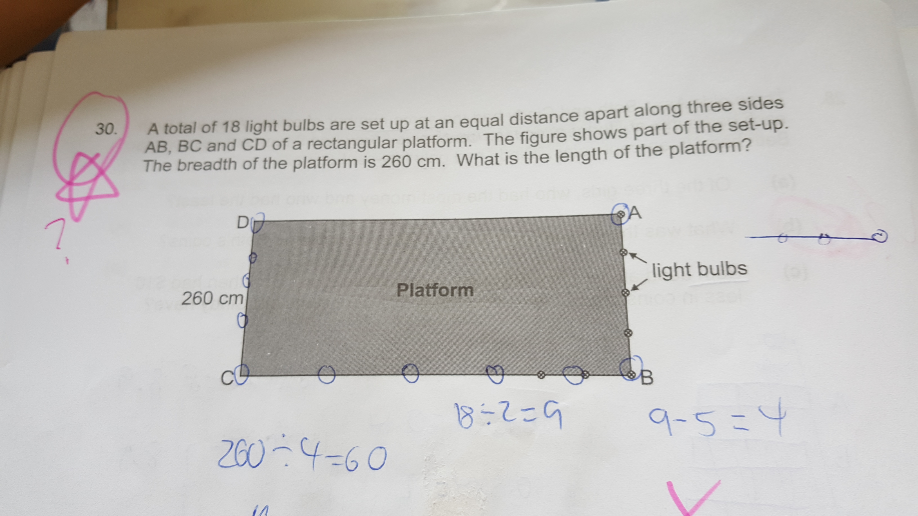# QuestionNumber of gaps between 5 bulbs along AB = 4

260 cm ÷ 4 = 65 cm (Distance of 1 gap)

5 bulbs x 2 breadths = 10 bulbs

18 bulbs – 10 bulbs = 8 bulbs (Remaining bulbs to be placed along BC)

8 bulbs + 2 bulbs (at C and D) = 10 bulbs

Gaps between 10 bulbs = 9

9 gaps x 65 cm = 585 cm (Ans)

0 Replies 1 Like

The length between each interval:260/4=65

18 light bulbs make total 17 intervals, so the length of 3 sides of the platform: 65*17=1105

So we get the length of the platform :1105-260*2=585

But if the bulbs are formed in a rectangle isnt there 18 intervals

5 bulbs on each side ——- 4 intervals on each side

18 – (5 x 2) = 8 (bulbs left)

8 + 1 = 9 (intervals along the length of the platform)

9 + 8 = 17 intervals

0 Replies 0 Likes

260/4 = 65
the length of the platform is (18 – 5 x 2 + 1) x 65 = 585

Ans : 585 cm

0 Replies 0 Likes